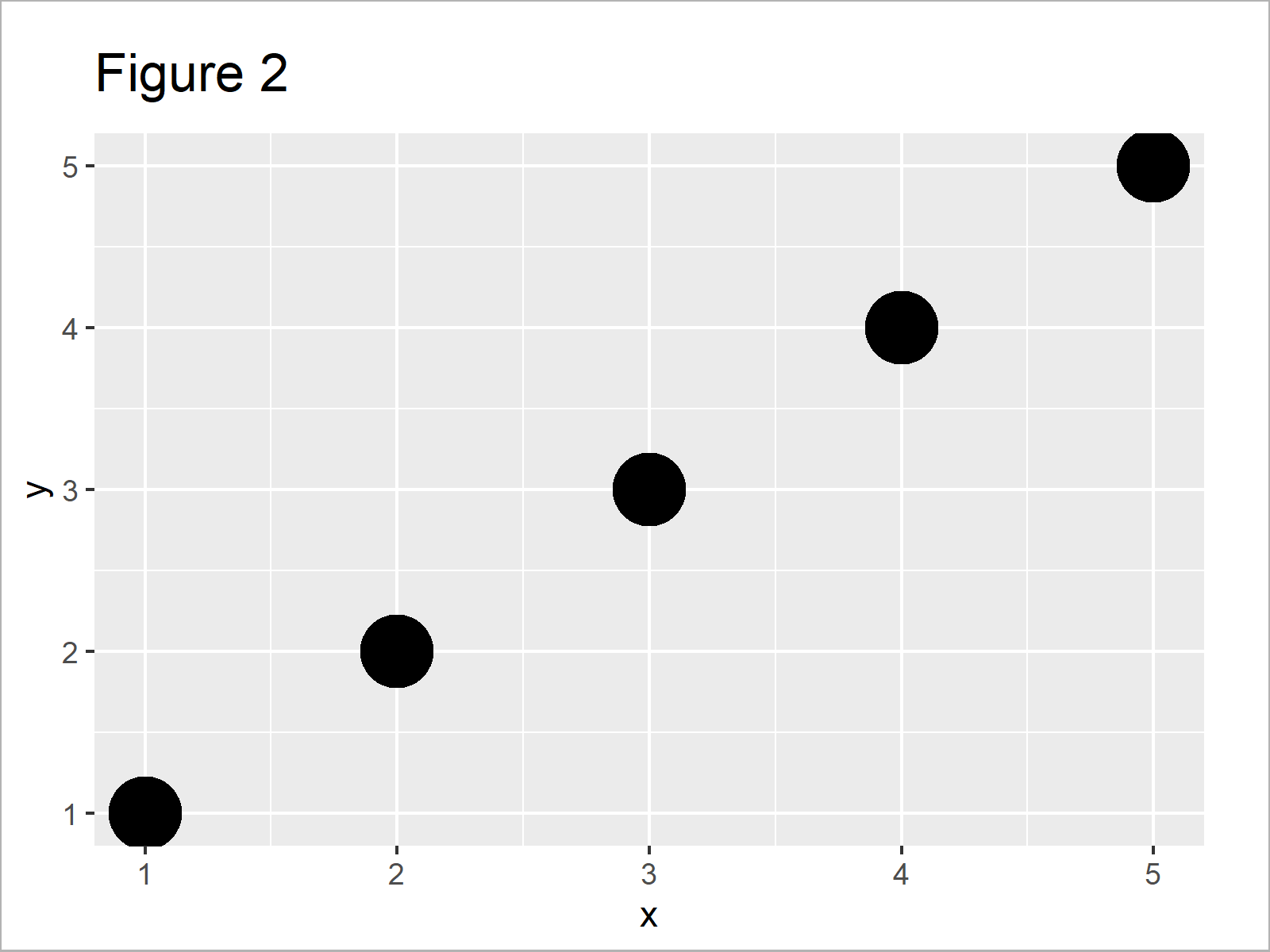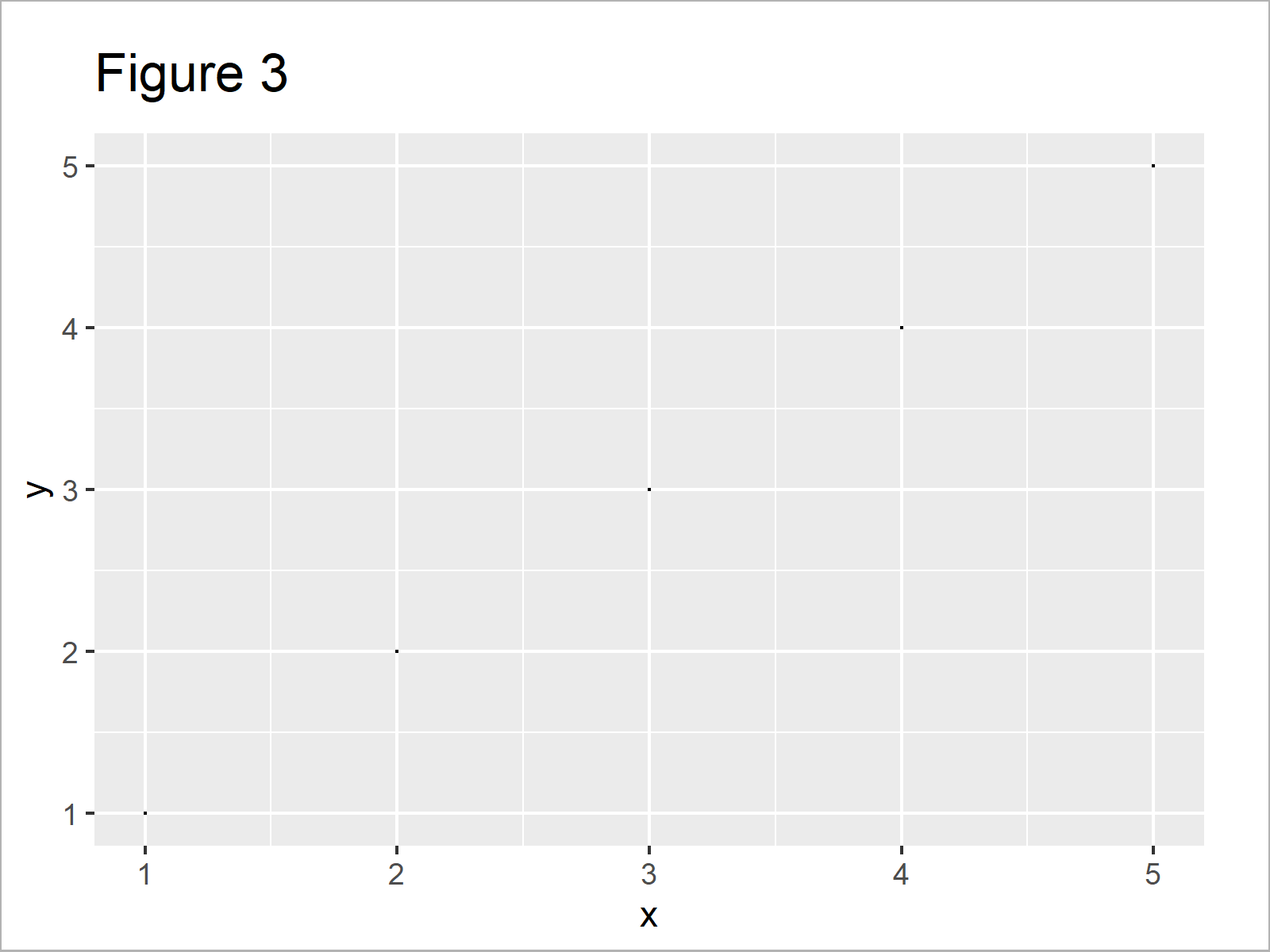# Change Point Size in ggplot2 Scatterplot in R (2 Examples)

In this article, I’ll demonstrate how to increase or decrease the size of points in a ggplot2 scatterplot in R.

Sound good? Let’s take a look at some R codes in action…

## Example Data, Packages & Basic Graphic

Consider the following example data.

```data <- data.frame(x = 1:5,        # Example data
y = 1:5)
data                               # Print example data```Table 1 shows that our example data is made of five rows and two integer columns called “x” and “y”.

We also need to install and load the ggplot2 package, if we want to use the corresponding functions:

```install.packages("ggplot2")        # Install ggplot2 package

Next, we can draw our data:

```ggplot(data, aes(x, y)) +          # Create basic ggplot2 plot
geom_point()```After running the previous R programming syntax the ggplot2 scatterplot shown in Figure 1 has been plotted.

## Example 1: Increase Point Size in ggplot2 Plot

The following code shows how to make the points in our ggplot2 scatterplot larger.

For this, we have to specify a large value to the size argument within the geom_point function.

Have a look at the following example code:

```ggplot(data, aes(x, y)) +          # Increase point size
geom_point(size = 10)```In Figure 2 it is shown that we have created a ggplot2 xy-plot with larger dots with the previous code.

## Example 2: Decrease Point Size in ggplot2 Plot

This example demonstrates how to create a ggplot2 scatterplot with tiny points.

For this, we have to specify a very small value to the size argument:

```ggplot(data, aes(x, y)) +          # Decrease point size
geom_point(size = 0.1)```Figure 3 shows the output of the previous code: A ggplot2 graphic with small points.

## Video & Further Resources

Have a look at the following video which I have published on my YouTube channel. I’m explaining the R programming code of this tutorial in the video.

Please accept YouTube cookies to play this video. By accepting you will be accessing content from YouTube, a service provided by an external third party.If you accept this notice, your choice will be saved and the page will refresh.

In addition, you could have a look at the other articles of this homepage. You can find a selection of articles on topics such as graphics in R, ggplot2, and labels below.

At this point you should know how to modify the point size of a ggplot2 graphic in R. If you have any additional questions, let me know in the comments.

Subscribe to the Statistics Globe Newsletter